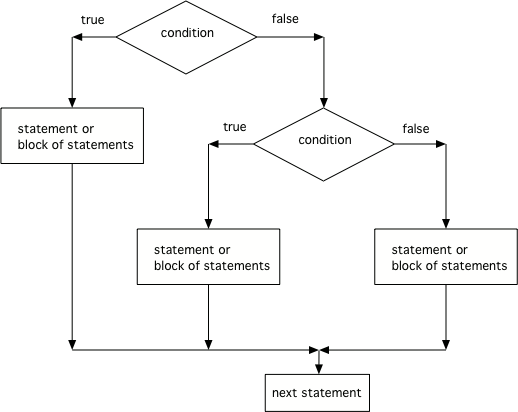5.2. Three or More Options¶

You can even pick between 3 or more possibilites. Just add else if for each possibility after the first if and before the last possibility, the else.Figure 1: The order that statements execute in a conditional with 3 options: if, else if, and else

Run the code below and try changing the value of x to get each of the three possible lines in the conditional to print.

Note

Another way to handle 3 or more conditional cases is to use the `switch` and `break` keywords, but these will not be on the exam. For a tutorial on using switch see https://docs.oracle.com/javase/tutorial/java/nutsandbolts/switch.html.

5-2-1: What does the following code print when x has been set to -5?

```if (x < 0) System.out.println("x is negative");
else if (x == 0) System.out.println("x is zero");
else System.out.println("x is positive");
```
• x is negative
• When x is equal to -5 the condition of x < 0 is true.
• x is zero
• This will only print if x has been set to 0. Has it?
• x is positive
• This will only print if x is greater than zero. Is it?

5-2-2: What does the following code print when x has been set to 2000?

```if (x < 0) System.out.println("x is negative");
else if (x == 0) System.out.println("x is zero");
else System.out.println("x is positive");
```
• x is negative
• This will only print if x has been set to a number less than zero. Has it?
• x is zero
• This will only print if x has been set to 0. Has it?
• x is positive
• The first condition is false and x is not equal to zero so the else will execute.

5-2-3: What does the following code print when x has been set to .8?

```if (x < .25) System.out.println("first quartile");
else if (x < .5) System.out.println("second quartile");
else if (x < .75) System.out.println("third quartile");
else System.out.println("fourth quartile");
```
• first quartile
• This will only print if x is less than 0.25.
• second quartile
• This will only print if x is greater than or equal to 0.25 and less than 0.5.
• third quartile
• The first only print if x is greater than or equal to 0.5 and less than 0.75.
• fourth quartile
• This will print whenever x is greater than 0.75.
Next Section - 5.3. Complex Conditionals